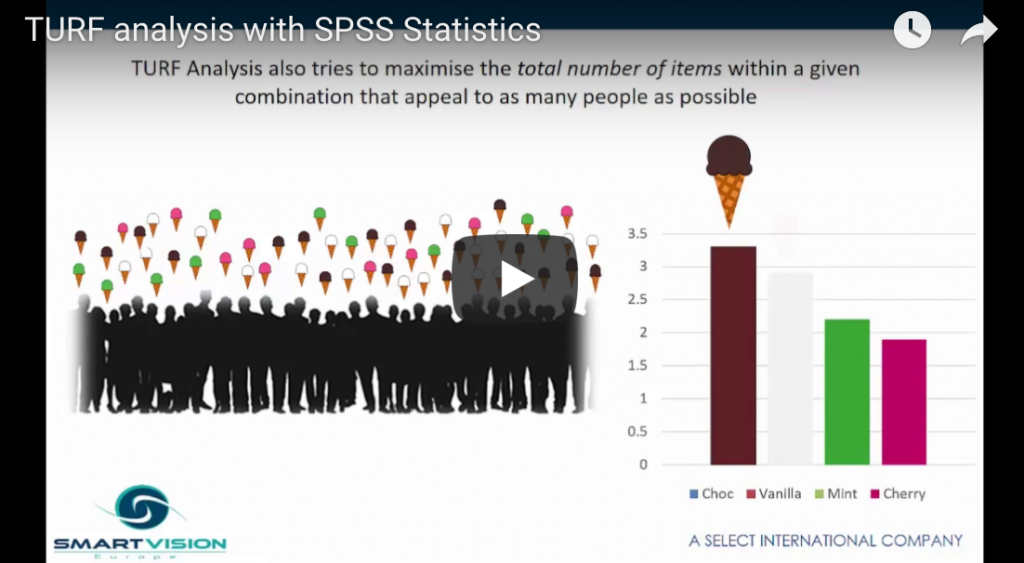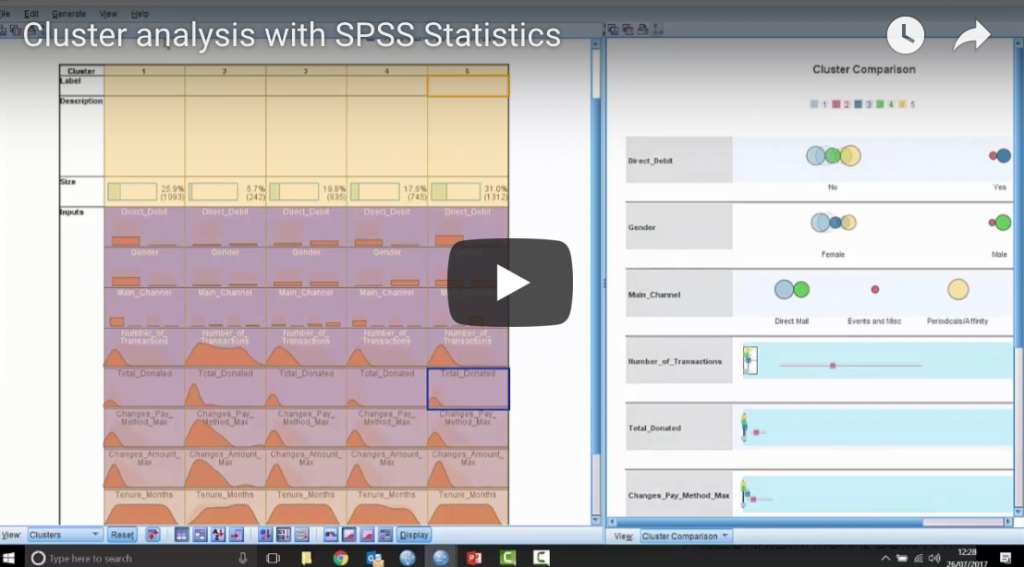# statistical techniques## TURF analysis with SPSS Statistics

In this video Jarlath Quinn introduces the popular TURF analysis technique and demonstrates how to apply it in IBM SPSS Statistics. TURF analysis is used in many industries to find the optimal sub-group of options from a wider portfolio in order to maximise their appeal to an audience or market. As such, TURF analysis is …## Cluster Analysis with IBM SPSS Statistics

In this video Jarlath Quinn explains what cluster analysis is, how it is applied in the real world and how easy it is create your own cluster analysis models in SPSS Statistics. The video includes: A demonstration of cluster analysis using sample data How to use the cluster viewer facility to interpret and make sense …

## Introduction to linear regression

In classical statistics, linear regression is regarded as  the ‘gateway to predictive modelling’. For decades students have been taught about regression from theory to practice simply because it still one of the most versatile and simple ways to understand and predict the effect of key factors on critical outcomes.   For instance, using regression you …